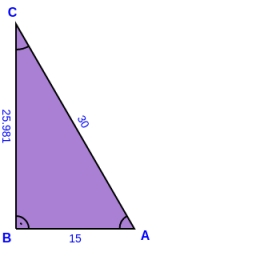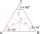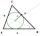# Right triangle

A circle with a radius of 5 cm is described in a right triangle with a 6 cm leg. What is the height at the hypotenuse of this triangle?

h =  4.8 cm

### Step-by-step explanation:Did you find an error or inaccuracy? Feel free to write us. Thank you!Tips to related online calculators
Pythagorean theorem is the base for the right triangle calculator.

#### You need to know the following knowledge to solve this word math problem:

We encourage you to watch this tutorial video on this math problem:

## Related math problems and questions:

• Right triangle eq2Hypotenuse of a right triangle is 9 cm longer than one leg and 8 cm longer than the second leg. Determine the circumference and area of a triangle.
• Right ΔA right triangle has the length of one leg 11 cm and the hypotenuse 61 cm size. Calculate the height of the triangle.
• The right triangleThe right triangle ABC has a leg a = 36 cm and an area S = 540 cm2. Calculate the length of the leg b and the median t2 to side b.
• HypotenuseCalculate the length of the hypotenuse of a right triangle if the length of one leg is 4 cm and its content area is 16 square centimeters.
• Inscribed circleXYZ is right triangle with right angle at the vertex X that has inscribed circle with a radius 5 cm. Determine area of the triangle XYZ if XZ = 14 cm.
• RT perimeterThe leg of the rectangular triangle is 7 cm shorter than the second leg and 8 cm shorter than the hypotenuse. Calculate the triangle circumference.
• Circle describedThe radius of the circle described to the right triangle with 6 cm long leg is 5 cm. Calculate the circumference of this triangle.
• Hexagonal pyramidCalculate the surface area of a regular hexagonal pyramid with a base inscribed in a circle with a radius of 8 cm and a height of 20 cm.
• Equilateral triangle vs circleFind the area of an equilateral triangle inscribed in a circle of radius r = 9 cm. What percentage of the circle area does it occupy?
• Euclid theoremsCalculate the sides of a right triangle if leg a = 6 cm, and a section of the hypotenuse, which is located adjacent to the second leg b is 5cm.
• Perimeter and legsDetermine the perimeter of a right triangle if the length of one leg is 75% length of the second leg and its content area is 24 cm2.
• Triangular prismThe base of the perpendicular triangular prism is a rectangular triangle with a hypotenuse of 10 cm and one leg of 8 cm. The prism height is 75% of the perimeter of the base. Calculate the volume and surface of the prism.
• Inscribed circleThe circle inscribed in a triangle has a radius of 3 cm. Express the area of the triangle using a, b, c.
• Cathethus and the inscribed circleIn a right triangle is given one cathethus long 14 cm and the radius of the inscribed circle of 5 cm. Calculate the area of this right triangle.
• Right triangleCalculate the missing side b and interior angles, perimeter, and area of ​​a right triangle if a=10 cm and hypotenuse c = 16 cm.
• Ratio of sidesCalculate the area of a circle with the same circumference as the circumference of the rectangle inscribed with a circle with a radius of r 9 cm so that its sides are in ratio 2 to 7.
• Quarter circleWhat is the radius of a circle inscribed in the quarter circle with a radius of 100 cm?